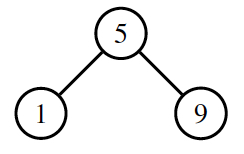# Homework 5

Due: Friday, March 15, 2019
Points: 100

A binary tree is a data structure that stores information in sorted order. The main component of a binary tree is a node. The node holds the data, and also two other nodes, called left and right. The data in the left node precedes that of the containing node, and the data in the right node follows that of the containing node. The left and right nodes in turn also can have left and right nodes, and so forth.

Here is an example of a binary tree containing the integers 1, 5, and 9.When we add 2 to the tree, it looks like this:And adding 7 and 10 creates this tree:Notice that as each new number is inserted into the tree, it is placed in an order (called inorder). To figure out where to put the new number, look at the root node. If the number in there is less than the one you want to insert, then go right (looking at the paper); if it’s greater, go left. Continue in this way until you reach the end of the tree (the node is called a leafnode). Then add a new node with the number in it; if it is less than the number in the leaf node, it goes on the left; if it’s greater, it goes on the right.

Your assignment is to write a Python program implementing a binary tree to count and sort the words in a file.

As before, we will proceed in stages. You need to turn in one program though, so these steps are to help you approach the program.

1. First, design the Node class. Your nodes should have four attributes: the data (a word in the file), a count (the number of times the word occurs), and the left and right nodes. You should also define methods for getting attributes of a node, setting attributes, and making a string from the contents of a node. For the latter, print the word, then the number of occurrences, like this:
```boy (3)
everybody (1)
word (12)
```

2. Next, write a recursive routine to print the tree. Begin at the root. Print the tree positioned to the left (it’s called the left subtree of the root, then the data in the root, then the right subtree of the root. Print a subtree in the same way: start at the root of the subtree, print the left subtree of the root, then the contents of the root, then the right subtree. If there is no subtree, skip printing it. Also, every time you recurse, add 2 blanks in front of the line you print.

3. Finally, write a program that reads input one line at a time, breaks it into words (which are maximal length strings of letters, numbers, and underscores “_”), and inserts each word into the tree, using the methods you defined. To do this, go down the tree, comparing the string to the data in each node. If the string precedes the data, look at the left subtree. If there is no node there, create one and put the word in there. Otherwise, repeat this, looking in the node at the root of the left subtree. Similarly, if the string follows the data, look at the right subtree. If there is no node there, create one and put the word in there. Otherwise, repeat this, looking in the node at the root of the right subtree. Finally, if the string and the data are the same, add 1 to the count and stop.

At the end, print the tree using the function you defined above.

Here is an example. The input

```Little Bo Peep
Has lost her sheep
And doesn't know where to find them.
Leave them alone!
They'll come home.
Wagging their tales behind them.
```
produces the output:
```    And (1)
Bo (1)
Has (1)
Leave (1)
Little (1)
Peep (1)
They (1)
Wagging (1)
alone (1)
behind (1)
come (1)
doesn (1)
find (1)
her (1)
home (1)
know (1)
ll (1)
lost (1)
sheep (1)
t (1)
tales (1)
their (1)
them (3)
to (1)
where (1)
```
For other examples, look at the files “alice.txt” and “listu.txt”. Your program should produce the output in “alice-out.txt” and “listu-out.txt”, respectively.Matt Bishop Office: 2209 Watershed Science Phone: +1 (530) 752-8060 Email: mabishop@ucdavis.edu